# 二、图像二值化处理流程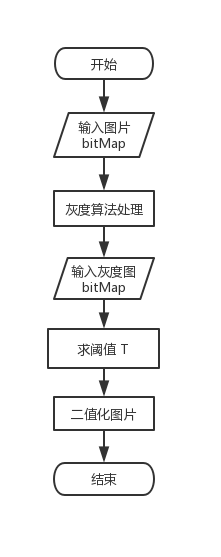# 三、以前的解决方案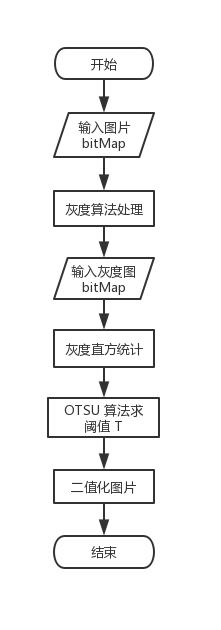Gray = R * 0.299 + G * 0.587 + B * 0.114

Gray = (R * 38 + G * 75 + B * 15) >> 7

``````/**
获取灰度图

@param bit_map 图像像素数组地址（ ARGB 格式）
@param width 图像宽
@param height 图像高
@return 灰度图像素数组地址
*/
int * gray_image(int *bit_map, int width, int height) {
double pixel_total = width * height; // 像素总数
if (pixel_total == 0) return NULL;
// 灰度像素点存储
int *gray_pixels = (int *)malloc(pixel_total * sizeof(int));
memset(gray_pixels, 0, pixel_total * sizeof(int));
int *p = bit_map;
for (u_int i = 0; i < pixel_total; i++, p++) {
// 分离三原色及透明度
u_char alpha = ((*p & 0xFF000000) >> 24);
u_char red = ((*p & 0xFF0000) >> 16);
u_char green = ((*p & 0x00FF00) >> 8);
u_char blue = (*p & 0x0000FF);

u_char gray = (red*38 + green*75 + blue*15) >> 7;
if (alpha == 0 && gray == 0) {
gray = 0xFF;
}
gray_pixels[i] = gray;
}
return gray_pixels;
}
``````

OTSU 算法：

OTSU 算法也称最大类间差法，有时也称之为大津算法，由大津于 1979 年提出，被认为是图像分割中阈值选取的最佳算法，计算简单，不受图像亮度和对比度的影响，因此在数字图像处理上得到了广泛的应用。它是按图像的灰度特性，将图像分成背景和前景两部分。因方差是灰度分布均匀性的一种度量，背景和前景之间的类间方差越大，说明构成图像的两部分的差别越大，当部分前景错分为背景或部分背景错分为前景都会导致两部分差别变小。因此，使类间方差最大的分割意味着错分概率最小。

``````ω0 = N0 / M × N							(1)
ω1 = N1 / M × N							(2)
N0 + N1 = M × N							(3)
ω0 + ω1 = 1								(4)
μ = ω0 * μ0 + ω1 * μ1					(5)
g = ω0 * (μ0 - μ)^2 + ω1 * (μ1 - μ)^2	(6)
``````

``````g = ω0 * ω1 * (μ0 - μ1)^2 　　 		(7)
``````

1、获取原图像的灰度图

2、灰度直方统计

``````/**
OTSU 算法获取二值图

@param bit_map 图像像素数组地址（ ARGB 格式）
@param width 图像宽
@param height 图像高
@param T 存储计算得出的阈值
@return 二值图像素数组地址
*/
int * binary_image_with_otsu_threshold_alg(int *bit_map, int width, int height, int *T) {

double pixel_total = width * height; // 像素总数
if (pixel_total == 0) return NULL;

unsigned long sum1 = 0;  // 总灰度值
unsigned long sumB = 0;  // 背景总灰度值
double wB = 0.0;        // 背景像素点比例
double wF = 0.0;        // 前景像素点比例
double mB = 0.0;        // 背景平均灰度值
double mF = 0.0;        // 前景平均灰度值
double max_g = 0.0;     // 最大类间方差
double g = 0.0;         // 类间方差
u_char threshold = 0;    // 阈值
double histogram = {0}; // 灰度直方图，下标是灰度值，保存内容是灰度值对应的像素点总数

// 获取灰度直方图和总灰度
int *gray_pixels = (int *)malloc(pixel_total * sizeof(int));
memset(gray_pixels, 0, pixel_total * sizeof(int));
int *p = bit_map;
for (u_int i = 0; i < pixel_total; i++, p++) {
// 分离三原色及透明度
u_char alpha = ((*p & 0xFF000000) >> 24);
u_char red = ((*p & 0xFF0000) >> 16);
u_char green = ((*p & 0x00FF00) >> 8);
u_char blue = (*p & 0x0000FF);

u_char gray = (red*38 + green*75 + blue*15) >> 7;
if (alpha == 0 && gray == 0) {
gray = 0xFF;
}
gray_pixels[i] = gray;

// 计算灰度直方图分布，Histogram 数组下标是灰度值，保存内容是灰度值对应像素点数
histogram[gray]++;
sum1 += gray;
}

// OTSU 算法
for (u_int i = 0; i < 256; i++)
{
wB = wB + histogram[i]; // 这里不算比例，减少运算，不会影响求 T
wF = pixel_total - wB;
if (wB == 0 || wF == 0)
{
continue;
}
sumB = sumB + i * histogram[i];
mB = sumB / wB;
mF = (sum1 - sumB) / wF;
g = wB * wF * (mB - mF) * (mB - mF);
if (g >= max_g)
{
threshold = i;
max_g = g;
}
}

for (u_int i = 0; i < pixel_total; i++) {
gray_pixels[i] = gray_pixels[i] <= threshold ? 0xFF000000:0xFFFFFFFF;
}

if (T) {
*T = threshold;    // OTSU 算法阈值
}

return gray_pixels;
}
``````

iPhone 6: imageSize：260, 260； OTSU 使用时间：0.005254； 5 次异步处理使用时间：0.029240

iPhone 6: imageSize：620, 284； OTSU 使用时间：0.029476； 5 次异步处理使用时间：0.050313

iPhone 6: imageSize：2560,1440； OTSU 使用时间：0.200595； 5 次异步处理使用时间：0.684509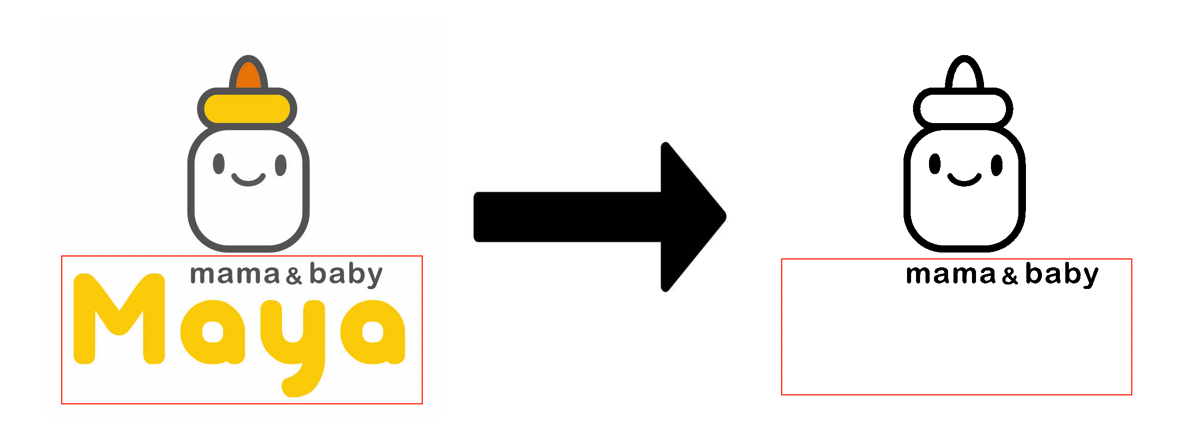• 存在问题总结
• 算法单一，对于不同图片处理结果可能与预期不一致
• 每次打印都对图片进行处理，没有缓存机制

# 四、新的解决方案

## 4.1 问题一 （算法单一，对于不同图片处理结果可能与预期不一致）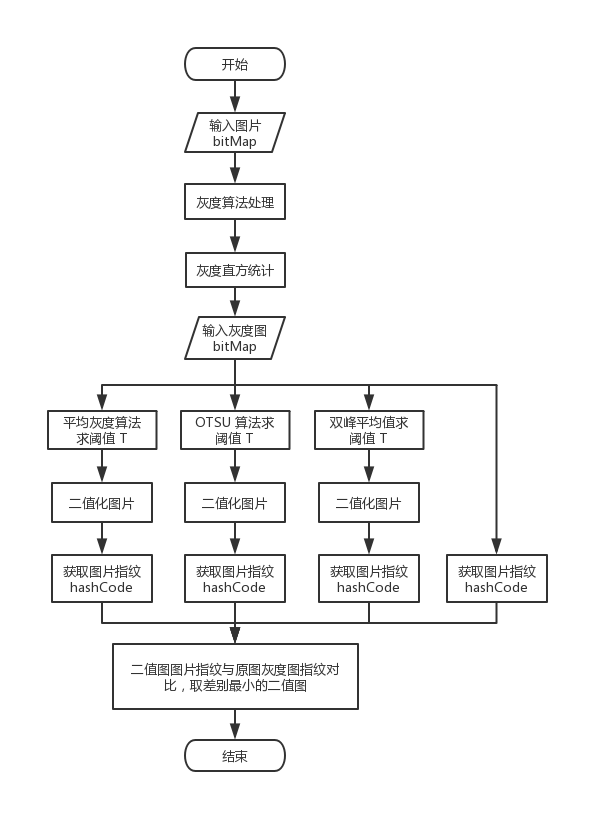T = sum / pixel_total

``````/**
平均灰度算法获取二值图

@param bit_map 图像像素数组地址（ ARGB 格式）
@param width 图像宽
@param height 图像高
@param T 存储计算得出的阈值
@return 二值图像素数组地址
*/
int * binary_image_with_average_gray_threshold_alg(int *bit_map, int width, int height, int *T) {

double pixel_total = width * height; // 像素总数
if (pixel_total == 0) return NULL;

unsigned long sum = 0;  // 总灰度
u_char threshold = 0;    // 阈值

int *gray_pixels = (int *)malloc(pixel_total * sizeof(int));
memset(gray_pixels, 0, pixel_total * sizeof(int));
int *p = bit_map;
for (u_int i = 0; i < pixel_total; i++, p++) {
// 分离三原色及透明度
u_char alpha = ((*p & 0xFF000000) >> 24);
u_char red = ((*p & 0xFF0000) >> 16);
u_char green = ((*p & 0x00FF00) >> 8);
u_char blue = (*p & 0x0000FF);

u_char gray = (red*38 + green*75 + blue*15) >> 7;
if (alpha == 0 && gray == 0) {
gray = 0xFF;
}
gray_pixels[i] = gray;
sum += gray;
}
// 计算平均灰度
threshold = sum / pixel_total;

for (u_int i = 0; i < pixel_total; i++) {
gray_pixels[i] = gray_pixels[i] <= threshold ? 0xFF000000:0xFFFFFFFF;
}

if (T) {
*T = threshold;
}

return gray_pixels;
}
``````

1、获取原图像的灰度图

2、灰度直方统计

3、平滑直方图

4、求双峰平均值作为阈值 T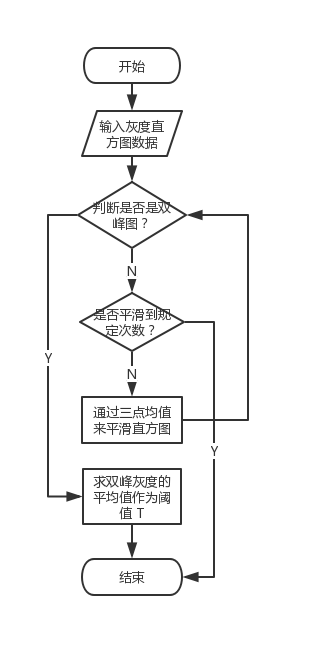``````// 判断是否是双峰直方图
int is_double_peak(double *histogram) {
// 判断直方图是存在双峰
int peak_count = 0;
for (int i = 1; i < 255; i++) {
if (histogram[i - 1] < histogram[i] && histogram[i + 1] < histogram[i]) {
peak_count++;
if (peak_count > 2) return 0;
}
}
return peak_count == 2;
}

/**
双峰平均值算法获取二值图

@param bit_map 图像像素数组地址（ ARGB 格式）
@param width 图像宽
@param height 图像高
@param T 存储计算得出的阈值
@return 二值图像素数组地址
*/
int * binary_image_with_average_peak_threshold_alg(int *bit_map, int width, int height, int *T) {
double pixel_total = width * height; // 像素总数
if (pixel_total == 0) return NULL;

// 灰度直方图，下标是灰度值，保存内容是灰度值对应的像素点总数
double histogram1 = {0};
double histogram2 = {0}; // 求均值的过程会破坏前面的数据，因此需要两份数据
u_char threshold = 0;    // 阈值

// 获取灰度直方图
int *gray_pixels = (int *)malloc(pixel_total * sizeof(int));
memset(gray_pixels, 0, pixel_total * sizeof(int));
int *p = bit_map;
for (u_int i = 0; i < pixel_total; i++, p++) {
// 分离三原色及透明度
u_char alpha = ((*p & 0xFF000000) >> 24);
u_char red = ((*p & 0xFF0000) >> 16);
u_char green = ((*p & 0x00FF00) >> 8);
u_char blue = (*p & 0x0000FF);

u_char gray = (red*38 + green*75 + blue*15) >> 7;
if (alpha == 0 && gray == 0) {
gray = 0xFF;
}
gray_pixels[i] = gray;

// 计算灰度直方图分布，Histogram数组下标是灰度值，保存内容是灰度值对应像素点数
histogram1[gray]++;
histogram2[gray]++;
}

// 如果不是双峰，则通过三点求均值来平滑直方图
int times = 0;
while (!is_double_peak(histogram2)) {
times++;
if (times > 1000) {				// 这里使用 1000 次，考虑到过多次循环可能会存在性能问题
return NULL;                          // 似乎直方图无法平滑为双峰的，返回错误代码
}
histogram2 = (histogram1 + histogram1 + histogram1) / 3;                   // 第一点
for (int i = 1; i < 255; i++) {
histogram2[i] = (histogram1[i - 1] + histogram1[i] + histogram1[i + 1]) / 3;       // 中间的点
}
histogram2 = (histogram1 + histogram1 + histogram1) / 3;           // 最后一点
memcpy(histogram1, histogram2, 256 * sizeof(double));                                  // 备份数据，为下一次迭代做准备
}

// 求阈值T
int peak = {0};
for (int i = 1, y = 0; i < 255; i++) {
if (histogram2[i - 1] < histogram2[i] && histogram2[i + 1] < histogram2[i]) {
peak[y++] = i;
}
}
threshold = (peak + peak) / 2;

for (u_int i = 0; i < pixel_total; i++) {
gray_pixels[i] = gray_pixels[i] <= threshold ? 0xFF000000:0xFFFFFFFF;
}

if (T) {
*T = threshold;
}

return gray_pixels;
}
``````

iPhone 6: imageSize：260, 260； average_peak 使用时间：0.035254

iPhone 6: imageSize：800, 800； average_peak 使用时间：0.101282

1、图片缩小尺寸一般缩小到 8 * 8 ，一共 64 个像素点。

2、将缩小的图片转换成灰度图。

3、计算灰度图的平均灰度。

4、灰度图的每个像素点的灰度与平均灰度比较。大于平均灰度，记为 1 ；小于平均灰度，记为 0。

5、计算哈希值，第 4 步的结果可以构成一个 64 为的整数，这个 64 位的整数就是该图片的指纹 hashCode 。

6、对比不同图片生成的指纹 hashCode ，计算两个 hashCode 的 64 位中有多少位不一样，即“汉明距离”，差异越少图片约相近。

1、图片缩小尺寸可自定义（必须是整数），但是最小像素数要为 64 个，也就是 width * height >= 64 。建议为 64 的倍数，为了减少误差。

5、哈希值不是一个 64 位的整数，而是一个存储 64 位整数的数组，数组的长度就是像素点数量对 64 的倍数（取最大的整数倍）。这样每生成一个 64 位的 hashCode 就加入到数组中，该数组就是图片指纹。

6、对比不同指纹时，遍历数组，对每一个 64 为整数进行对比不同位数，最终结果为，每一个 64 位整数的不同位数总和。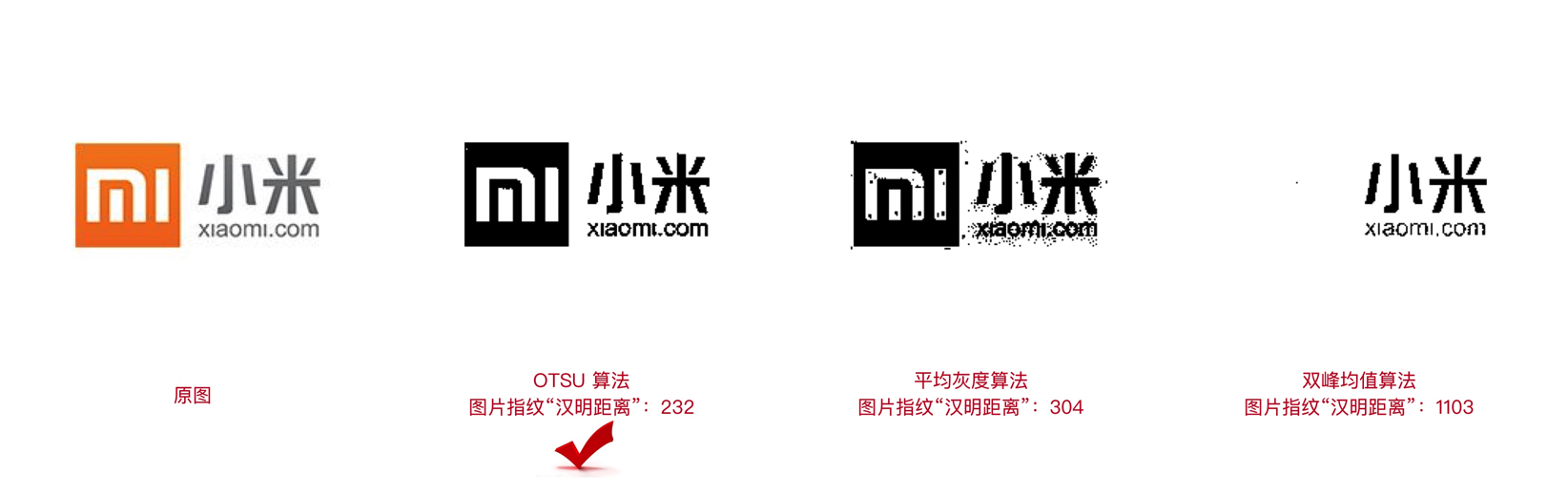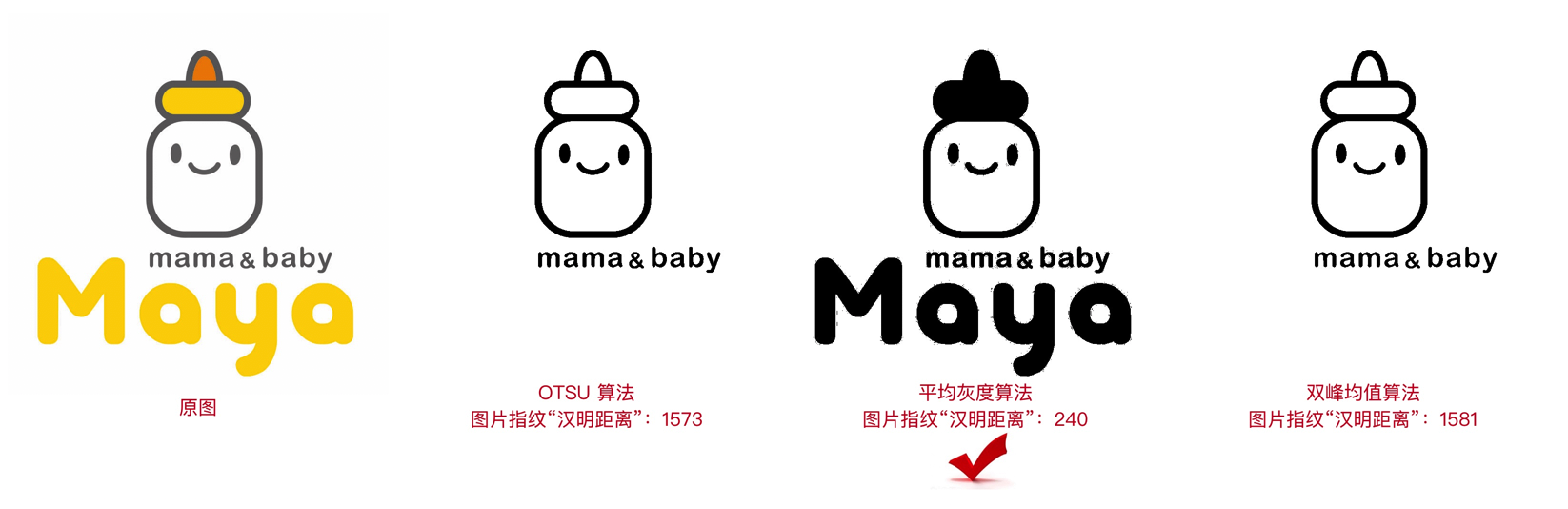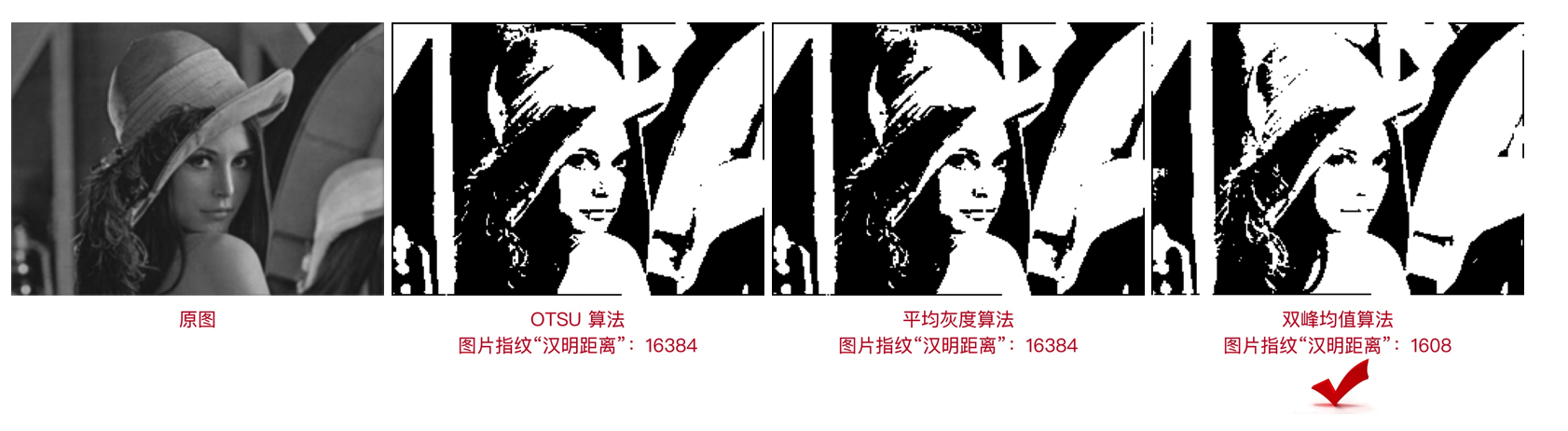## 4.2 问题二 （每次打印都对图片进行处理，没有缓存机制）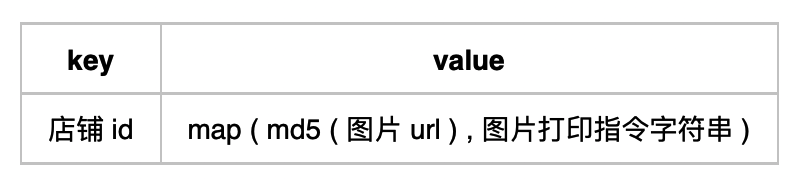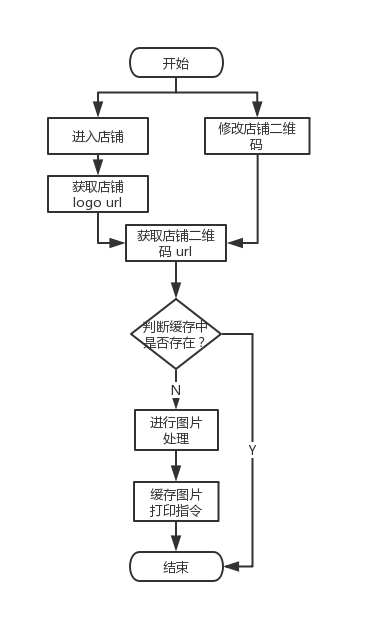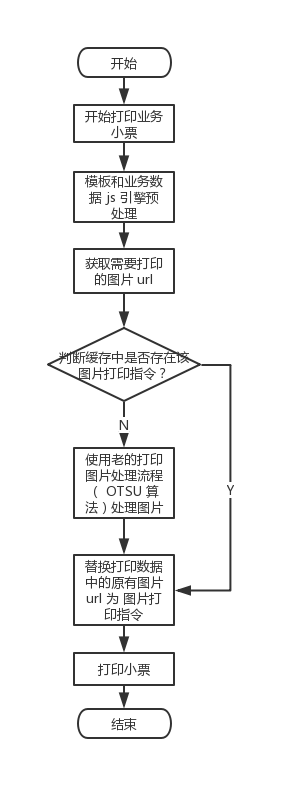# 五、未来期望与规划

• 添加新流程处理统计，对商家 logo 和店铺二维码处理后的最优算法进行统计，为后续优化做数据准备。
• 处理后的结果如果商家不满意，商家可以自主选择处理二值图的阈值 T ，达到满意为止。
• 图片更新不及时问题，PC 后台修改了图片无法及时更新本地缓存。
• 图片精细化处理，针对二维码可以采用分块处理算法。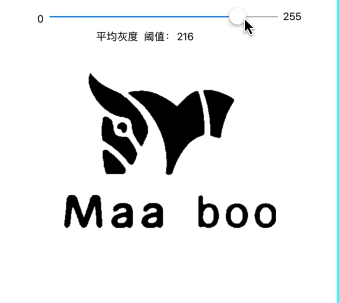#参考链接
- 灰度计算公式, by zyl910
- 大津算法, by wiki
- 图像处理算法, by laviewpbt